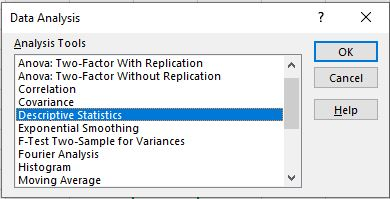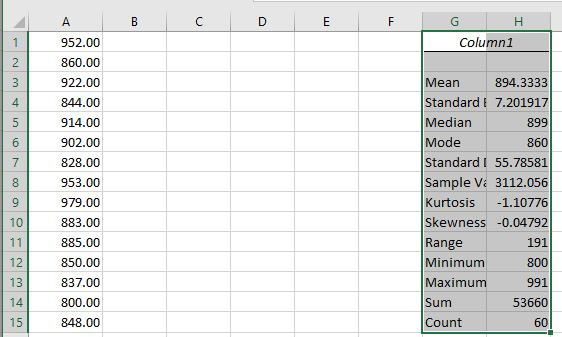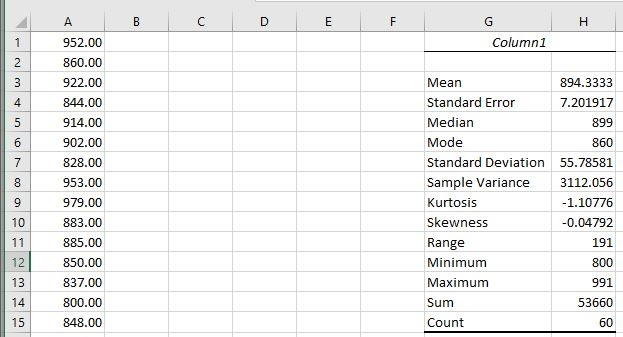# 2.05 Using the Data Analysis Tool to generate Descriptive Statistics

$$\newcommand{\vecs}{\overset { \rightharpoonup} {\mathbf{#1}} }$$ $$\newcommand{\vecd}{\overset{-\!-\!\rightharpoonup}{\vphantom{a}\smash {#1}}}$$$$\newcommand{\id}{\mathrm{id}}$$ $$\newcommand{\Span}{\mathrm{span}}$$ $$\newcommand{\kernel}{\mathrm{null}\,}$$ $$\newcommand{\range}{\mathrm{range}\,}$$ $$\newcommand{\RealPart}{\mathrm{Re}}$$ $$\newcommand{\ImaginaryPart}{\mathrm{Im}}$$ $$\newcommand{\Argument}{\mathrm{Arg}}$$ $$\newcommand{\norm}{\| #1 \|}$$ $$\newcommand{\inner}{\langle #1, #2 \rangle}$$ $$\newcommand{\Span}{\mathrm{span}}$$ $$\newcommand{\id}{\mathrm{id}}$$ $$\newcommand{\Span}{\mathrm{span}}$$ $$\newcommand{\kernel}{\mathrm{null}\,}$$ $$\newcommand{\range}{\mathrm{range}\,}$$ $$\newcommand{\RealPart}{\mathrm{Re}}$$ $$\newcommand{\ImaginaryPart}{\mathrm{Im}}$$ $$\newcommand{\Argument}{\mathrm{Arg}}$$ $$\newcommand{\norm}{\| #1 \|}$$ $$\newcommand{\inner}{\langle #1, #2 \rangle}$$ $$\newcommand{\Span}{\mathrm{span}}$$$$\newcommand{\AA}{\unicode[.8,0]{x212B}}$$

## Generating Descriptive Statistics

Using the Desktop version of Excel, enter the data into the spreadsheet.  Once the data is in the Excel spreadsheet, click on the Data tab on the main menu, and select Data Analysis.Click on the Data Analysis Option on the far right-hand side of the menu.  A dialog box will appear.Select Descriptive Statistics and hit the OK button.  A new dialog box will appear that asks for the location of the data, where the results will be put, and what information to include in the results.The results that will be generated will be put in the spreadsheet starting at cell G1.  The result will look like the picture below.  The results, include the Mean, Standard Error, Median, Mode, Standard Deviation, Sample Variance, Kurtosis, Skewness, Range, Minimum, Maximum, Sum of value, and Count of numbers.To increase the column width of column G, click on G and select the Home tab.  Then select Cells group, select Format, and click on AutoFit Column Width.## Quartiles

To get the quartiles, you will use the Excel function =QUARTILE.INC(DATA LIST, k) where k is defined below.

 K Measurement 0 Minimum 1 First Quartile 2 Median 3 Third Quartile 4 Maximum

To get the first quartile, Q1, enter Q1 in cell G16.  Then enter the formula in cell H16, =QUARTILE.INC(A1:A60, 1) then hit the Enter key.  Next, enter Q3 in cell G17.  Next, enter the formula =QUARTILE.INC(A1:A60, 3) then hit the Enter key.

## Inter-quartile Range

In cell G18, enter IQR.  In cell H18, enter = H17 - H16.

## Outliers using Quartile data

In cell G19, enter Q1 - 1.5*IQR.  In cell H19 enter the formula, = H16 - 1.5*H18.  In cell G20, enter Q3-1.5*IQR. In cell H20 enter the formula, =H17 - 1.5*H20.

## Empirical Rule

In cell G21, enter Empirical Rule.  In cell H21, Lower Limit.  In cell I21, Upper Limit.
In cell G22, enter 68% of the data.  In cell H22, enter the formula, =H3 - H7.  In cell I22, enter the formula, =H3+H7.
In cell G23, enter 95% of the data.  In cell H23, enter the formula, =H3-2*H7.  In cell I23, enter the formula, =H3+2*H7.
In cell G24, enter 99.7% of the data.  In cell H24, enter the formula, =H3-3*H7.  In cell I24, enter the formula, =H3+3*H7.

## Video

View the video below to understand how to generate descriptive statistics in Excel using the Data Analysis Toolpak.

2.05 Using the Data Analysis Tool to generate Descriptive Statistics is shared under a not declared license and was authored, remixed, and/or curated by LibreTexts.